# Can A Circle Be A Function? (3 Important Ideas)

Circles come up often in mathematics and in our lives.  The question is, how does the concept of a function relate to a circle?

So, can a circle be a function?  A circle cannot be a function, since it fails the vertical line test for the vertical line going through its center.  However, the top (or bottom) half of a circle is a function, since each input has only one output.  A quarter of a circle can give us a one-to-one function that is its own inverse.

Of course, a function can give us the top or bottom half of a circle.  We can use two functions together to describe an entire circle.

In this article, we’ll talk about circles and how we can describe them with multiple functions.  We’ll also take a look at the inverse of an equation that describes part of a circle.

Let’s get going.

## Can A Circle Be A Function?

A circle cannot be a function, since it fails the vertical line test.  That is, we can draw a vertical line that intersects the graph of a circle more than once.

Specifically, the vertical line through the center of the circle will intersect the circle twice: once at the top of the circle, and once at the bottom of the circle.

Remember that for the circle with equation

• (x – a)2 + (y – b)2 = R2,

the center is (a, b) and the radius is R.  So:

• the top of the circle is R units above the center, at the point (a, b + R)
• the bottom of the circle is R units below the center, at the point (a, b – R)

So the vertical line x = a (through the center of the circle) will intersect the circle at (a, b + R) and (a, b – R).

The example below will help to explain the idea further.

### Example: Vertical Line Test For A Circle

Let’s say we have the circle

• (x – 2)2 + (y – 5)2 = 16,

which is a circle with center (2, 5) and a radius of 4 (since 16 = 42).  So a = 2, b = 5, and R = 4.

If we draw the vertical line x = 2 through the center of the circle, it will intersect the circle at two points:

• the top of the circle, at the point (a, b + R) = (2, 5 + 4) = (2, 9).
• the bottom of the circle, at the point (a, b – R) = (2, 5 – 4) = (2, 1).

We can also find these two points directly from the circle equation with x = 2:

• (x – 2)2 + (y – 5)2 = 16  [circle equation]
• (2 – 2)2 + (y – 5)2 = 16  [substitute x = 2, the vertical line through the center]
• (y – 5)2 = 16  [(2 – 2)2 = 0]
• y – 5 = +/- 4  [take the square root on both sides, and take both positive and negative]
• y = 5 +/- 4
• y = 9, 1

Since the graph of this relation fails the vertical line test, it is not a function. (In fact, no full circle is a function).

You can see a graph below.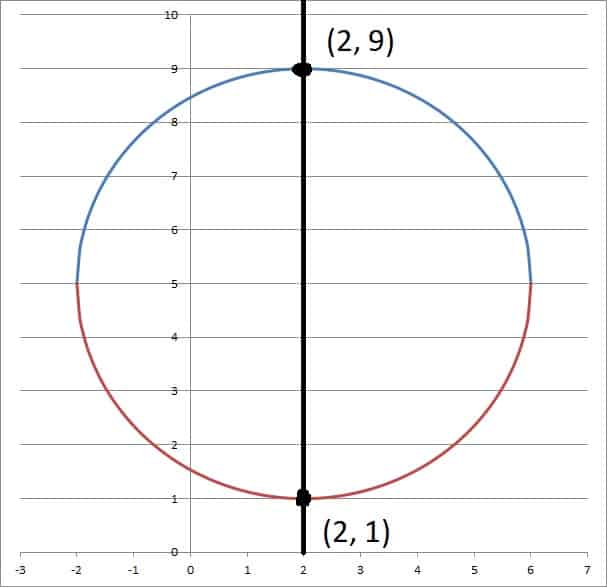Here we can see that the circle fails the vertical line test, since the line x = 2 passes through the circle at two points: (2, 1) and (2, 9).

## Can A Semi Circle Be A Function?

A semi-circle can be a function if we take the top half or bottom half of the circle (0 to 180 degrees or 180 to 360 degrees).  To do this, we solve for y in the circle equation.

This will involve taking a square root.  When we do this, we choose either the positive or negative root to give us one half of the circle (positive for the top half, negative for the bottom half).

Here is how we solve for y in the circle equation:

• (x – a)2 + (y – b)2 = R2  [circle equation]
• (y – b)2 = R2 – (x – a)2  [isolate y terms]
• (y – b) = +/-S  [where S is the square root of R2 – (x – a)2]
• y = b +/- S

Let’s say we want to take the top half of the circle to make our function.  Then we would choose the positive part of the square root to give us the function

• y = b + S or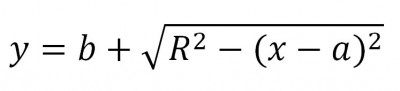Here we have the top half of the circle equation solved for y, which is a function.

The graph of this equation is the top half of a circle.  As such, it no longer fails the vertical line test, and so the relation is actually a function:

• f(x) = b + S

However, note that this function is not one-to-one, since it fails the horizontal line test (that is, it has repeating y values).  The horizontal line y = b will intersect the semi-circle twice: once at (a – R, b) and once at (a + R, b).

### Example: Semi Circle Function

Continuing with the example from earlier, remember that our circle has the following parameters:

• a = 2
• b = 5
• R = 4

Using the function from above

• f(x) = b + S, or

with a = 2, b = 5, and R = 4, we have the semi-circle function: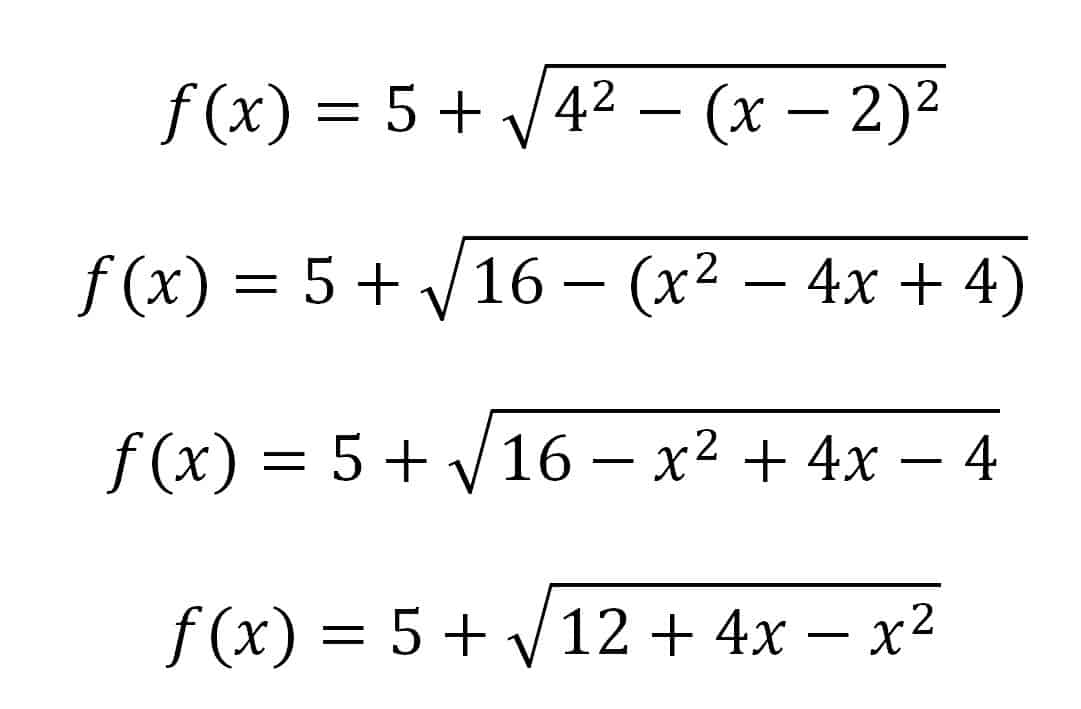Here is the semi circle function with a = 2, b = 5, and R = 4.

You can see a graph below.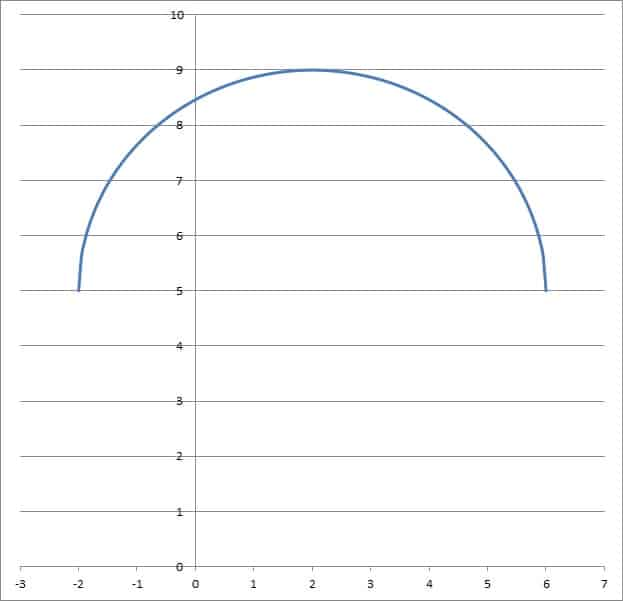The graph of the semi circle function with a = 2, b = 5, and R = 4.

## Can A Quarter Circle Be A One-To-One Function?

A quarter circle can be a one-to-one function if we take a quadrant of the circle, which means one of these:

• top right (0 to 90 degrees)
• top left (90 to 180 degrees)
• bottom left (180 to 270 degrees)
• bottom right (270 to 360 degrees)

Each of these quadrants is a one-to-one function, with the same equation as the semi-circle:

• f(x) = b + S,

but with a domain restriction (the domain consists of only half of the x-values, on either the left or right side of the semi-circle).

Since the quarter circle is a one-to-one function, it is invertible.  In fact, the function is its own inverse, meaning

f-1(x) = b + S

If we solve the equation for x and then switch x and y, we will see that we get the exact same equation as the original circle.

You can see the graph of the quarter circle function below: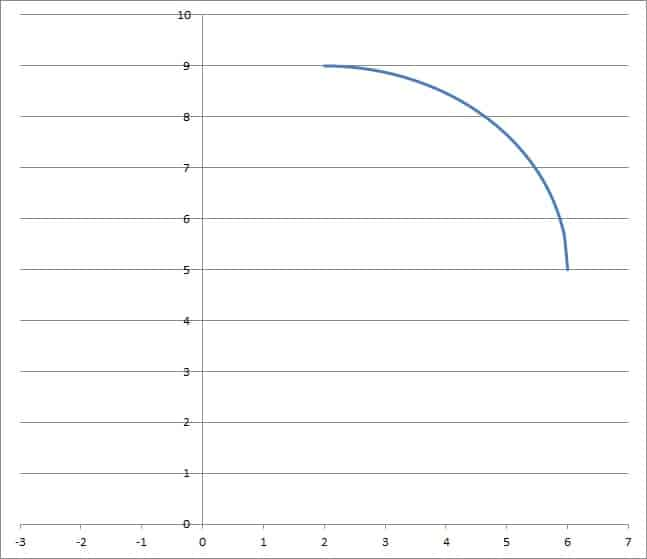The graph of the quarter circle function with a = 2, b = 5, and R = 4. This function is one-to-one due to the domain restriction to [2, 6].

## Conclusion

Now you know about how the concept of a function relates to circles.  You also know how to turn part of a circle into a function or even a one-to-one function.

To learn about applications of circles, check out my article on how circles are used.

You might also want to read my article on common questions about ellipses (a circle is just a specific type of ellipse).